# Game Lab: Dino Jump

## Make a Game in the Hack Club Game Lab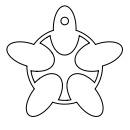@leomcelroy

Let's make a game in the Hack Club Game Lab!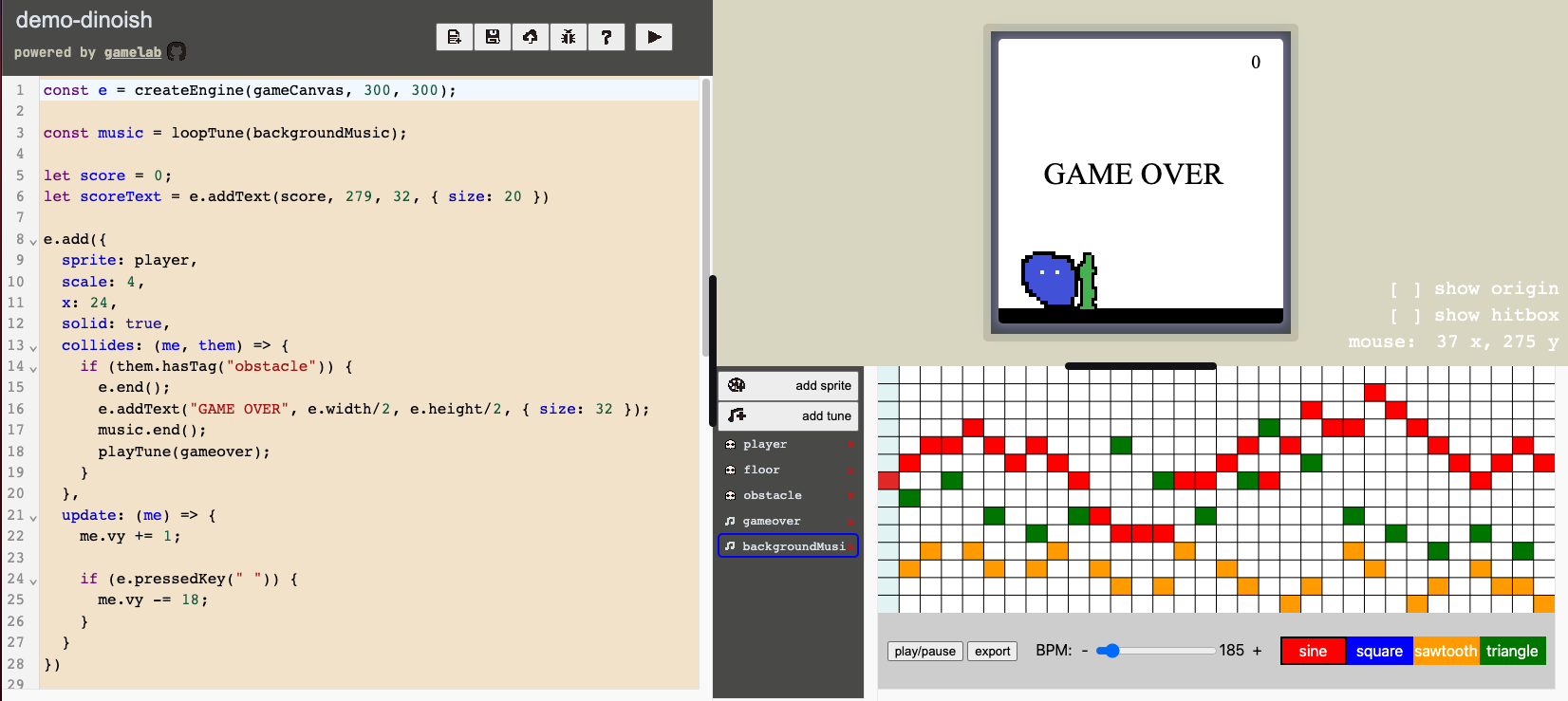## Walkthrough

Start by creating a new project. It's the first button on the left in the button menu.

``````const e = createEngine(gameCanvas, 300, 300);

// your code goes in here

e.start();
``````

We are then going to add a character.

First we need to make a sprite asset in the sprite editor.

We can add our character by using the `add` property on the engine.

You'll have to rename the sprite to match the name in the game script on the line that looks like `sprite: player`.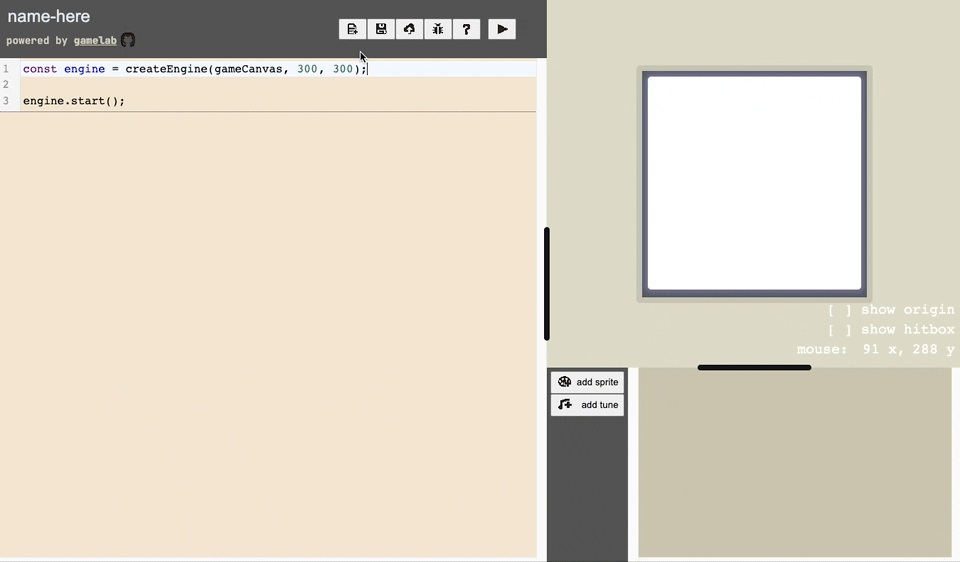``````
sprite: player,
scale: 4, // we can size up the sprite with scale
x: 40, // position it with x and y
})
``````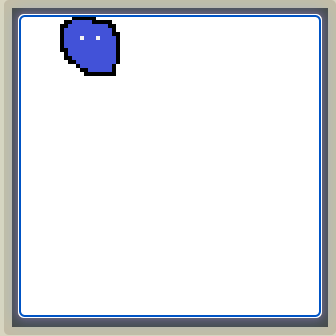`add` takes a lot of optional parameters which you can find in the reference pop-out.

We want our sprite to fall so let's add some gravity. We can do so by adding an update function which will execute each step in the game loop.

``````
sprite: player,
scale: 4, // we can size up the sprite with scale
x: 40, // position it with x and y
update: (me) => { // this will run each step in the game loop
me.vy += 1; // velocity changing every frame is acceleration
}
})
``````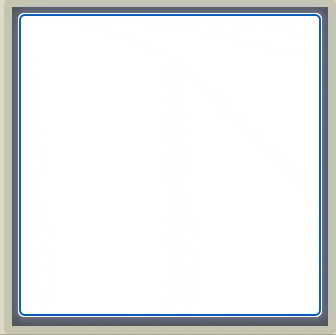Now our character just falls into oblivion. Let's prevent that by adding a floor.

Create a floor sprite like we did before with our player sprite and add it to the game script.

``````e.add({
sprite: player,
scale: 4, // we can size up the sprite with scale
x: 40, // position it with x and y
update: (me) => { // this will run each step in the game loop
me.vy += 1; // velocity changing every frame is acceleration
}
})

sprite: floor,
scale: 15,
x: 0,
y: 284,
})
``````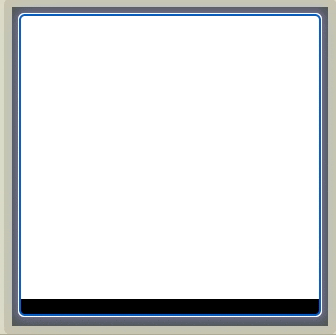We've got a floor now but our character falls through it? We need to let the engine know that the player and the floor should not be able to pass through each other. We can do that with the `solid` property.

``````e.add({
solid: true,
sprite: player,
scale: 4, // we can size up the sprite with scale
x: 40, // position it with x and y
update: (me) => { // this will run each step in the game loop
me.vy += 1; // velocity changing every frame is acceleration
}
})

solid: true,
sprite: floor,
scale: 15,
x: 0,
y: 284,
})
``````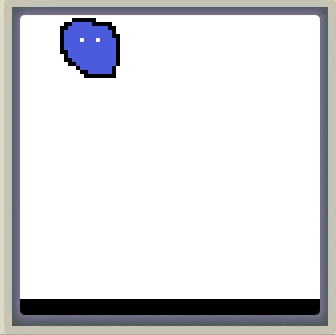Let's give our player the ability to jump.

We can do this with the `pressedKey` property of the engine. We'll check if the key was pressed in the updated loop of the player.

``````e.add({
solid: true,
sprite: player,
scale: 4, // we can size up the sprite with scale
x: 40, // position it with x and y
update: (me) => { // this will run each step in the game loop
me.vy += 1; // velocity changing every frame is acceleration

if (e.pressedKey(" ")) { // if the space key was pressed
me.vy -= 20; // we jump
}
}
})
``````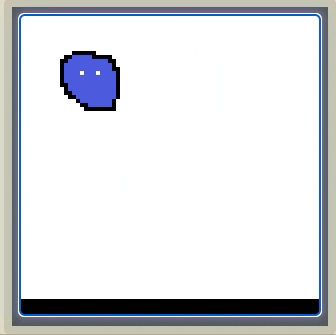Now that we've got a character and some controls let's create some obstacles.

We can draw an obstacle sprite like we did before and pop it into the game script.

``````e.add({
sprite: obstacle,
scale: 3,
x: 250,
y: 225
})
``````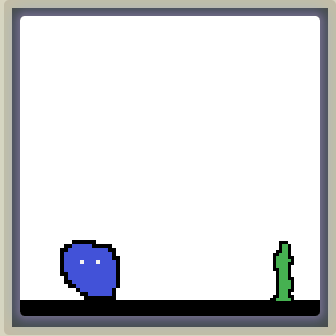We want this obstacle to move to the left so let's give it some velocity.

``````e.add({
sprite: obstacle,
scale: 3,
x: 250,
y: 225,
vx: -3
})
``````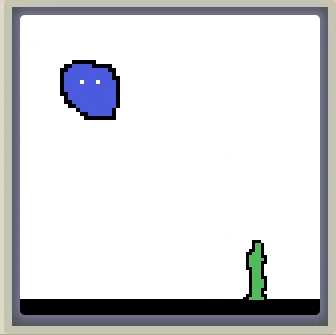When we hit the player the game should end. To do that let's add a `collides` handler to the player object. To check what we collided with we'll need to add a tag to the obstacle.

``````e.add({
solid: true,
sprite: player,
scale: 4, // we can size up the sprite with scale
x: 40, // position it with x and y
update: (me) => { // this will run each step in the game loop
me.vy += 1; // velocity changing every frame is acceleration

if (e.pressedKey(" ")) { // if the space key was pressed
me.vy -= 20; // we jump
}
},
collides: (me, them) => {
if (them.hasTag("obstacle")) { // did we hit the obstacle
e.end(); // then end the game
}
}
})

tags: ["obstacle"],
sprite: obstacle,
scale: 3,
x: 250,
y: 225,
vx: -3
})
``````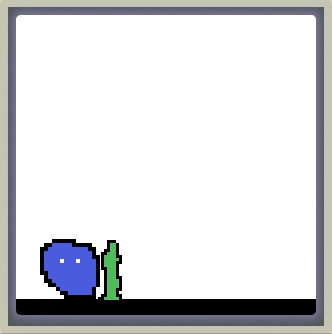While we are at it let's add some text to spruce up the game ending.

``````e.add({
solid: true,
sprite: player,
scale: 4, // we can size up the sprite with scale
x: 40, // position it with x and y
update: (me) => { // this will run each step in the game loop
me.vy += 1; // velocity changing every frame is acceleration

if (e.pressedKey(" ")) { // if the space key was pressed
me.vy -= 20; // we jump
}
},
collides: (me, them) => {
if (them.hasTag("obstacle")) { // did we hit the obstacle
e.end(); // then end the game

// place "GAME OVER" in the center of the screen
e.addText("GAME OVER", e.width/2, e.height/2, { size: 32 });
}
}
})
``````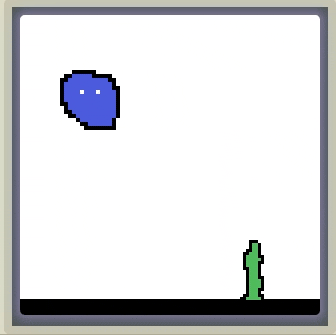But if we jump over the obstacle then we need to remove it.

``````e.add({
tags: ["obstacle"],
sprite: obstacle,
scale: 3,
x: 250,
y: 225,
vx: -3,
update: (me) => {
// if my right side is off the
// left hand side of the screen
if (me.x + me.width < 0) {
e.remove(me); // remove me from the game
}
}
})
``````

But we also need a new obstacle so let's wrap the whole obstacle adding into a function. We'll also need to call it to initialize our obstacles.

``````const addObstacle = () => {
tags: ["obstacle"],
sprite: obstacle,
scale: 3,
x: e.width,
y: 225,
vx: -3,
update: (me) => {
// if my right side is off the
// left hand side of the screen
if (me.x + me.width < 0) {
e.remove(me); // remove me from the game
}
}
})
}

``````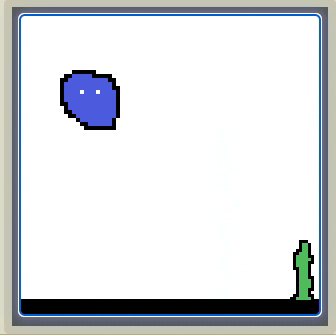This game would get boring because the obstacles are always the same. Let's add some randomness to make it more interesting.

``````const addObstacle = () => {

// generate a random number between 2 and 5
const speed = Math.random()*(5-2)+2;

tags: ["obstacle"],
sprite: obstacle,
scale: 3,
x: e.width,
y: 225,
vx: -speed,
update: (me) => {
// if my right side is off the
// left hand side of the screen
if (me.x + me.width < 0) {
e.remove(me); // remove me from the game
}
}
})
}

``````

Let's add a score to keep track of how many obstacles we've cleared.

We'll initialize it below the engine declaration.

``````const e = createEngine(gameCanvas, 300, 300);

let score = 0;
let scoreText = e.addText(score, 279, 32, { size: 20 });
``````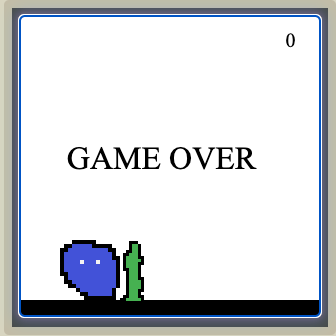Every time we clear an obstacle let's increment and redraw the score.

``````const addObstacle = () => {

// generate a random number between 2 and 5
const speed = Math.random()*(5-2)+2;

tags: ["obstacle"],
sprite: obstacle,
scale: 3,
x: e.width,
y: 225,
vx: -speed,
update: (me) => {
// if my right side is off the
// left hand side of the screen
if (me.x + me.width < 0) {
e.remove(me); // remove me from the game

score = score + 1; // add one to the score
scoreText.text = score; // reset the score text
}
}
})
}
``````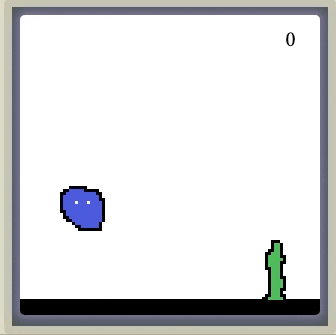Let's add some sound effects to the game as well.

We can create a tune in the asset editor.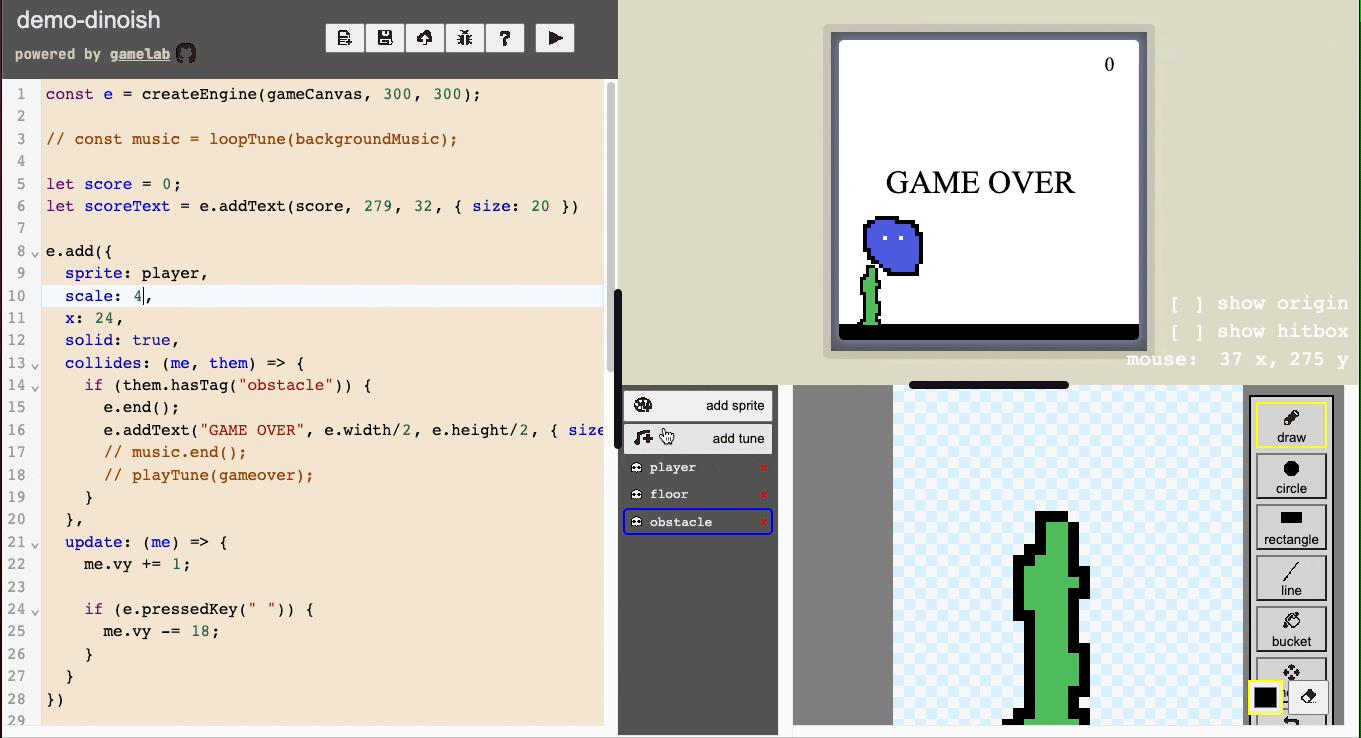To add it to the game we can use either `loopTune` or `playTune`.

Let's start the background music with the game.

``````const e = createEngine(gameCanvas, 300, 300);

// I'm assigning it to a variable so I can stop this later
const music = loopTune(backgroundMusic);

let score = 0;
let scoreText = e.addText(score, 279, 32, { size: 20 });
``````

When the game ends let's stop the background music and play a game over noise.

``````e.add({
solid: true,
sprite: player,
scale: 4, // we can size up the sprite with scale
x: 40, // position it with x and y
update: (me) => { // this will run each step in the game loop
me.vy += 1; // velocity changing every frame is acceleration

if (e.pressedKey(" ")) { // if the space key was pressed
me.vy -= 20; // we jump
}
},
collides: (me, them) => {
if (them.hasTag("obstacle")) { // did we hit the obstacle
e.end(); // then end the game

// place "GAME OVER" in the center of the screen
e.addText("GAME OVER", e.width/2, e.height/2, { size: 32 });

// stop the background music
music.end();

// play a game over sound effect
playTune(gameoverTune);
}
}
})
``````

## Complete Game

This is what it looks like all together. You can click here to play the game and see the assets.

And here is the complete code.

``````const e = createEngine(gameCanvas, 300, 300);

const music = loopTune(backgroundMusic);

let score = 0;
let scoreText = e.addText(score, 279, 32, { size: 20 })

sprite: player,
scale: 4,
x: 24,
solid: true,
collides: (me, them) => {
if (them.hasTag("obstacle")) {
e.end();
e.addText("GAME OVER", e.width/2, e.height/2, { size: 32 });
music.end();
playTune(gameover);
}
},
update: (me) => {
me.vy += 1;

if (e.pressedKey(" ")) {
me.vy -= 20;
}
}
})

sprite: floor,
scale: 15,
x: 0,
y: 284,
solid: true
})

const addObstacle = () => {

const speed = Math.random()*(5-2)+2;

tags: ["obstacle"],
sprite: obstacle,
scale: 3,
x: e.width,
y: 225,
vx: -speed,
update: (me) => {
if (me.x + me.width < 0) {
e.remove(me);
score = score + 1;
scoreText.text = score;
}
}
})
}

e.start();
``````

## Extensions

• Can you make the character only jump on the ground?
• Can you add sound effects to the jump?
• Can you turn it into a "Flappy Birds" style game?

## Help with Game Lab Development

The Hack Club Game Lab is all open-source and is actively developed by the Hack Club community. We welcome pull requests, bug reports, and feedback! If you want to join the conversation head over to the #gamelab-dev channel on the Hack Club Slack.